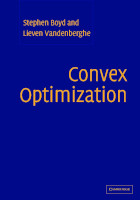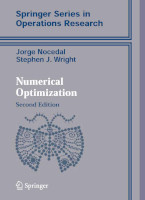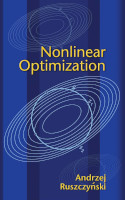## Intro

This site contains the description of the course Nonlinear Optimisation Algorithms (NOPT008) organized primarily for students of the Master's Computer Science program in Czech as well as English versions. This course is one of the Suggested Elective courses (Povinně volitelné in Czech) for specialization Optimization, see description in Czech or English. This course follows the previous one called Fundamentals of Nonlinear Optimization (NOPT018), which discusses the theoretical formulations of a nonlinear optimization problem and their properties.

## Description

Many real-world tasks are optimization in nature. The classic solution to such problems consists of the use of linear programming. In many cases, however, such a definition is imprecise, and even approximation techniques are not providing satisfactory results. In these cases, we usually adopt techniques of nonlinear programming. These techniques assume more complex structures of conditions and/or optimization functions.

In the entire nonlinear optimization task, several vital steps affect its success. In addition to the clever definition of the optimization problem, in terms of precision and computational complexity, another crucial aspect is using suitable optimization algorithms. Due to the more complex structure, algorithms in this area are typically iterative with decreasing estimation error and only sometimes guarantee convergence to the global optimum. This course deals not only with the construction of the methods but also their speed and convergence.

## Topics

The course consists of the following topics.

1. Introduction to nonlinear optimizations
• Examples of problems motivating nonlinear problems.
• Basic classification of optimization tasks.
• Definitions of some basic notions.
2. Convergence of iterative methods
• Introduction to convergence.
• Q-convergence and R-convergence and their relation.
• Quadratic, linear and superlinear convergence.
3. Gradient descent methods and linesearch
• Linesearch methods - Armijo, Goldstein, Wolfe.
• Satisfiability of the (strong) Wolfe conditions.
• Zoutendij theorem and its corrolaries.
• Convergence for strong convex functions with Lipschitz continuity.
5. Newton and trust region method
• Newton method and its convergence.
• Trust region methods.
• Sorensen Lemma and its consequences.
6. Trust region methods in detail
• Various strategies of step/direction selection in trust-region.
• Cauchy point and dogleg method.
• Correctness of dogleg method.
7. Quasi-newton methods
• Introduction to quasi-newton methods.
• Convergence of quasi-newton methods (Denis-Moré).
• Approximation of Hessian - DFP, (L-)BFGS, SR1.
• Principles of conjugate gradient (CG) method.
• Motivation on linear programming.
• Nonlinear version (Fletcher-Reeves, Polak-Ribiere).
9. Constraint optimization
• Introduction to constraint optimization.
• Methods based on KKT conditions.
• Active set and null-space methods.
10. Penalty and barier methods
• Principles of penalty and barier methods.
• Examples of methods.
• Characteristics and convergence of such methods.
11. Interior point methods
• Principles of Interior point methods (IPM) for linear programming.
• Extension of IPM to nonlinear case.
• Properties of IPM in context of nonlinear programming.

## Organization

The course is organized in lectures providing basic theory on topics given above. Seminars are based on solution of individual project.

Continuous evaluation is based on the result of individual project. Final evaluation consists of combined written and oral exam.

This section contains the list of references basic and advanced for the course, some of which are available online..

#### Basic referencesStephen Boyd and Lieven Vandenberghe Jorge Nocedal and Stephen Wright Andrzej Ruszczynski
Convex Optimization Numerical Optimization Nonlinear Optimization
Cambridge University Press, 2004 Springer, 2006 Princeton University Press, 2006

#### Relevant lecture notes